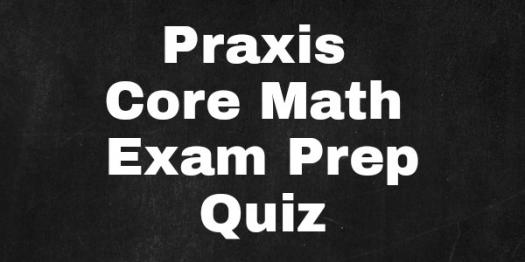# PRAXIS Core Math Exam Prep

10 Questions | Total Attempts: 116SettingsMathematics is the skeleton upon which all sciences are based. It is used to test, proof and attest the accuracy of a fact. Those who desire to teach this subject to be must sound, competent and do so with accuracy. The Praxis Core Math Exam contains apt questions which are to be answered within a period. Prepare diligently for this exam with this well prepared questions. Let's do this!

Related Topics
• 1.
What branch of mathematics focus figures with three sides and angles?
• A.

Logic

• B.

Set theory

• C.

Trigonometry

• D.

Geometry

• 2.
What branch of mathematics measure the degree of changes?
• A.

Geometry

• B.

Algebra

• C.

Statistics

• D.

Calculus

• 3.
When was set theory founded?
• A.

1867

• B.

1897

• C.

1876

• D.

1874

• 4.
What is the study of subsets of real line called?
• A.

Inner model theory

• B.

Combinatory set theory

• C.

Fuzzy set theory

• D.

Descriptive set theory

• 5.
What is the origin of the word "mathematics"?
• A.

Indian

• B.

Greek

• C.

Roman

• D.

Latin

• 6.
What is a cardinal number with extra property called?
• A.

Cardinal property

• B.

Cardinal integer

• C.

Integer

• D.

Large cardinal

• 7.
Who invented the method of forcing in set theory?
• A.

David Euler

• B.

Thomas Bidden

• C.

Richard Cantor

• D.

Paul Cohen

• 8.
What is value of angle on a straight line?
• A.

190°

• B.

120°

• C.

170°

• D.

180°

• 9.
Which of these does not involve the principles of trigonometry?
• A.

Pharmacy

• B.

Quantity survey

• C.

Medical imaging

• D.

Achitecture

• 10.
How many are the Pythagorean identities?
• A.

One

• B.

Four

• C.

Two

• D.

Three